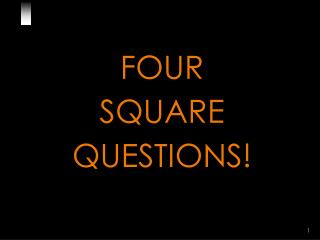Download PresentationFOUR SQUARE QUESTIONS!

# FOUR SQUARE QUESTIONS!

Download Presentation## FOUR SQUARE QUESTIONS!

- - - - - - - - - - - - - - - - - - - - - - - - - - - E N D - - - - - - - - - - - - - - - - - - - - - - - - - - -
##### Presentation Transcript

1. FOUR SQUARE QUESTIONS!

3. 4 SquareQuestions B A Q 1 Q 1 Divide the white area in square A into two equal pieces. Easy! Isn't it? D C

4. 4 SquareQuestions B A Q 1 Q 1 Divide the white area in square A into two equal pieces. Here is the answer! D C

5. 4 SquareQuestions B A Q 2 Q 2 Divide the white area in square B into three equal pieces. Not so difficult! D C

6. 4 SquareQuestions B A Q 2 Q 2 Divide the white area in squareBinto three equal pieces. Here is the answer! D C

7. 4 SquareQuestions B A Q 3 Q 3 Divide the white area in squareCinto four equal pieces. Very difficult? That's right! D C

8. 4 SquareQuestions B A Q 3 Q 3 Divide the white area in squareCinto four equal pieces. Here's the answer! D C

9. 4 SquareQuestions B A Be ready! Here comes the last question! D C

10. 4 SquareQuestions B A Q 4 Q 4 Divide the white area in squareDinto seven equal pieces. World Record is SEVEN seconds! D C

11. 4 SquareQuestions B A Q 4 Q 4 Divide the white area in squareDinto seven equal pieces. Any ideas? D C

12. 4 SquareQuestions B A Q 4 Q 4 Divide the white area in squareDinto seven equal pieces. Are you still puzzled? D C

13. 4 SquareQuestions B A Q 4 Q 4 Divide the white area in squareDinto seven equal pieces. Here’s the answer! D C

14. 4 SquareQuestions B A Q 4 Q 4 Divide the white area in squareDinto seven equal pieces. Was it really that tough? D C

15. 4 SquareQuestions B A Q 4 Q 4 Divide the white area in squareDinto seven equal pieces. If you didn't get the answer, it was just that your mind was conditioned to seek a complex solution! D C

16. 4 SquareQuestions • Lessons learnt: • Most of the times, our mind gets conditioned so much by the circumstances that we can not see the obvious. • When we go to a specialist doctor with a simple headache, diagnosis starts from migraine, upwards! • We assume that things are so complicated and we get entrapped in the ghost of it’s thoughts. • We carry on the same syndrome to our relationships and make them complicated.

17. BE SIMPLE IN LIFE ! THE GREATNESS OF THIS MAN WAS HIS SIMPLICITY!

18. HAVE A NICE DAY!